# 6 5 Linear Inequalities Day 1 Graphing Inequalities

• Slides: 116 -5: Linear Inequalities Day 1Graphing Inequalities in the Coordinate Plane • When a line is drawn in a plane, the line separates the plane into ________________. TWO distinct regions called HALF-PLANES BOUNDARY of the two regions. • The line itself is the ________ NOT belong in either half plane. • The boundary does _____ half-plane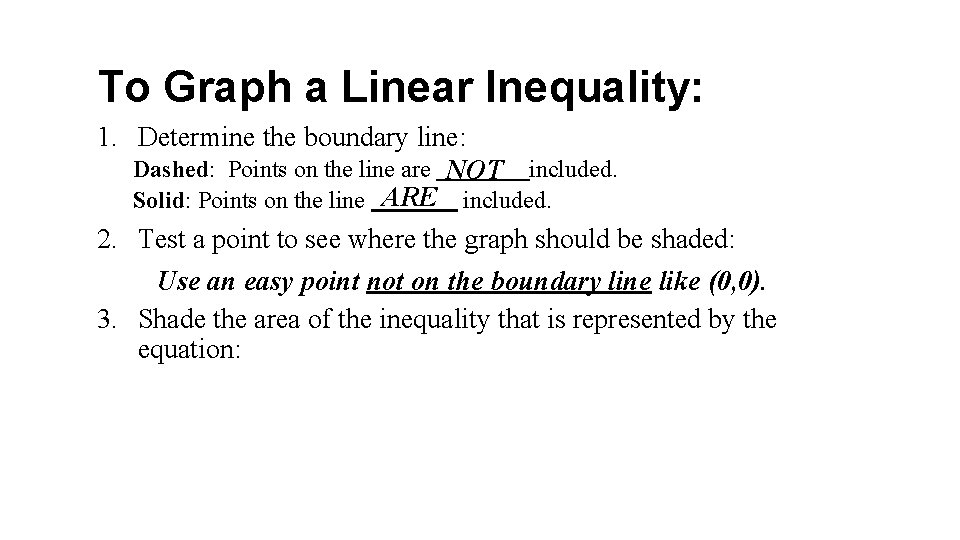To Graph a Linear Inequality: 1. Determine the boundary line: Dashed: Points on the line are NOT included. Solid: Points on the line ARE included. 2. Test a point to see where the graph should be shaded: Use an easy point not on the boundary line like (0, 0). 3. Shade the area of the inequality that is represented by the equation: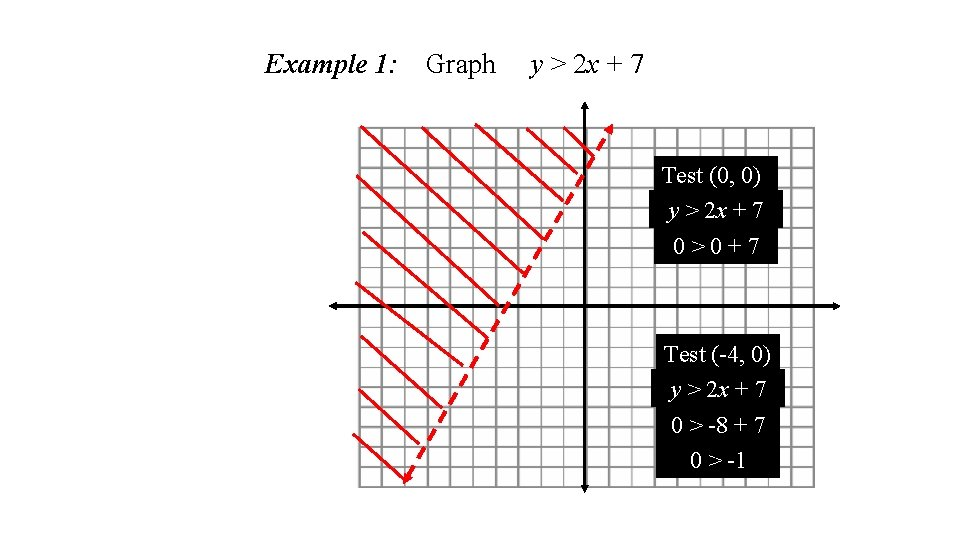Example 1: Graph y > 2 x + 7 Test (0, 0) y > 2 x + 7 0>0+7 Test (-4, 0) y > 2 x + 7 0 > -8 + 7 0 > -1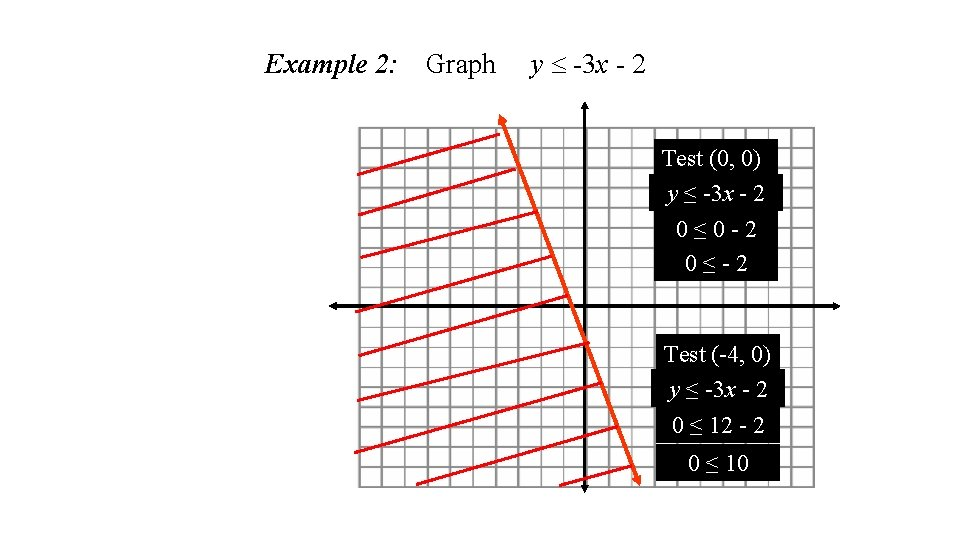Example 2: Graph y -3 x - 2 Test (0, 0) y ≤ -3 x - 2 0≤ 0 -2 0≤-2 Test (-4, 0) y ≤ -3 x - 2 0 ≤ 12 - 2 0 ≤ 10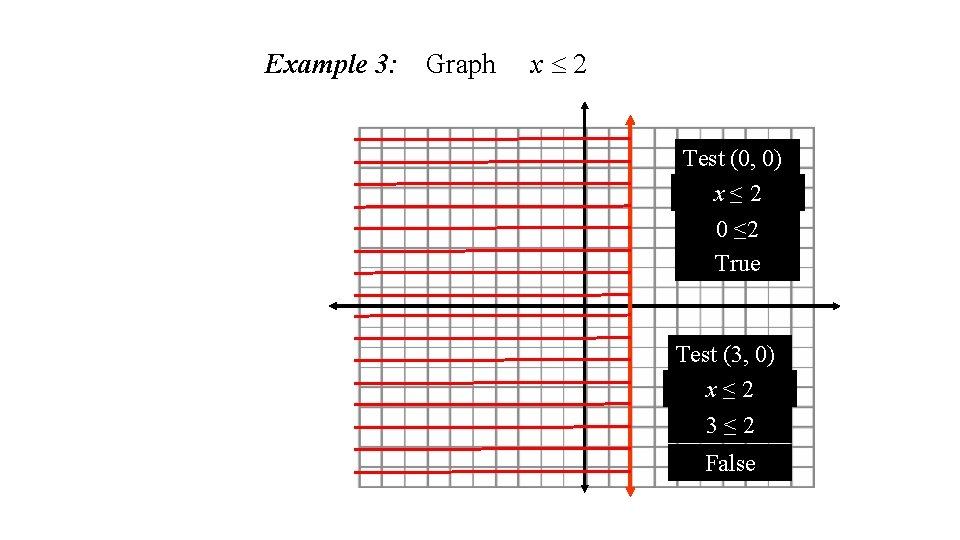Example 3: Graph x 2 Test (0, 0) x≤ 2 0 ≤ 2 True Test (3, 0) x≤ 2 3≤ 2 FalseHomework: p. 418 -419 # 6 -22 even6 -5: Linear Inequalities Day 2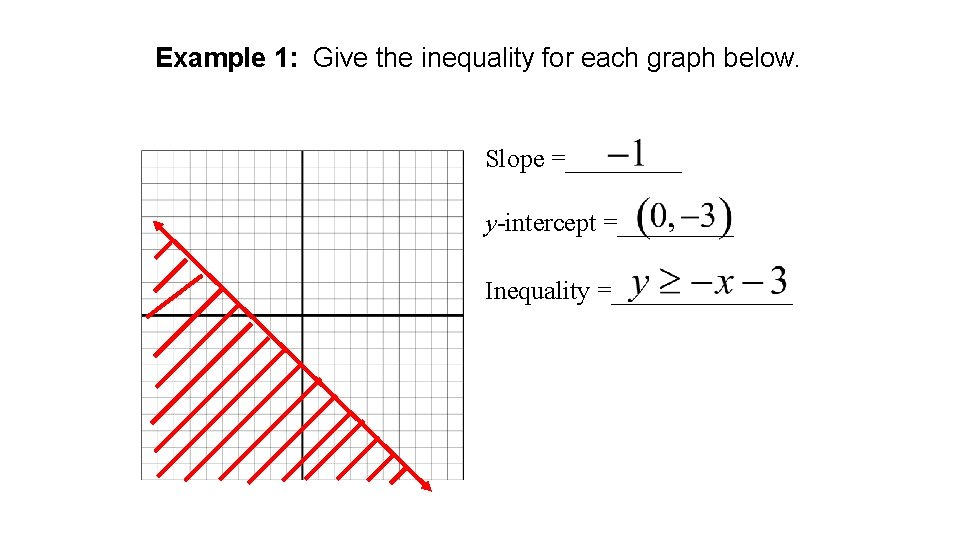Example 1: Give the inequality for each graph below. Slope =_____ y-intercept =_____ Inequality =_______Example 2: Give the inequality for each graph below. Inequality =_______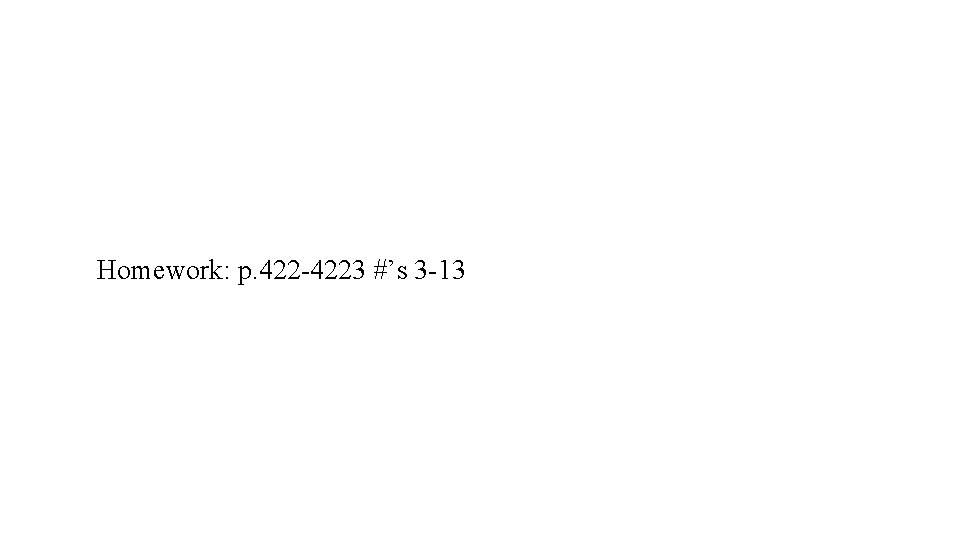Homework: p. 422 -4223 #’s 3 -13